• 2022-04-11 06:02:34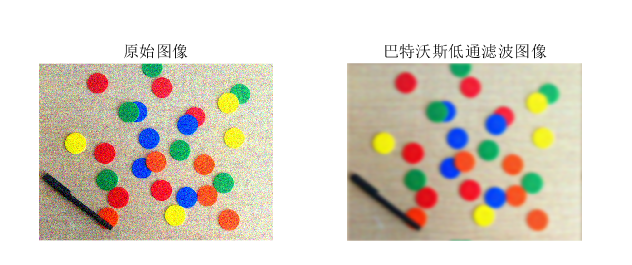clc,clear,close all % 清理命令区、清理工作区、关闭显示图形
warning off % 消除警告
feature jit off % 加速代码运行
D0 = 20; % 阻止的频率点与频域中心的距离
n = 2; % 阶次
R = imnoise(im(:,:,1),‘gaussian’,0,0.01); % R + 白噪声
G = imnoise(im(:,:,2),‘gaussian’,0,0.01); % G + 白噪声
B = imnoise(im(:,:,3),‘gaussian’,0,0.01); % B + 白噪声
im = cat(3,R,G,B); % 原图像 + 白噪声
R1 = freqfilter_btw_lp(R,D0,n); % 巴特沃斯低通滤波器
G1 = freqfilter_btw_lp(G,D0,n); % 巴特沃斯低通滤波器
B1 = freqfilter_btw_lp(B,D0,n); % 巴特沃斯低通滤波器
im1 = cat(3,R1,G1,B1);
figure(‘color’,[1,1,1])
subplot(121),imshow(im,[]); title(‘原始图像’)
subplot(122),imshow(im1,[]); title(‘巴特沃斯低通滤波图像’);
function im5 = freqfilter_btw_lp(im,D0,n)
if ~isa(im,‘double’)
im1 = double(im)/255;
end
im2 = fft2(im1); % 傅里叶变换
im3 = fftshift(im2); % 中心化

[N1, N2] = size(im3);
n1 = fix(N1 / 2);
n2 = fix(N2 / 2);
for i = 1:N1
for j = 2:N2
d = sqrt((i-n1)2+(j-n2)2);
h = 1/(1 + 0.414 * (d / D0)^(2*n)); % 巴特沃斯低通滤波器
result(i,j) = h * im3(i,j);
end
end
result = ifftshift(result); % 反中心化
im4 = ifft2(result); % 反变换
im5 = im2uint8(real(im4)); % 滤波图像

end

更多相关内容
• 巴特沃斯低通滤波matlab实现代码 DIP-Filter 1.概况 项目：实现一个通用的高通、低通、带通和带阻滤波器函数。其中又分别实现理想、巴特沃思和指数等滤波形式。用实现的函数对图1（lena_noise.bmp）进行低通处理，图...
• 采用matlab实现巴特沃斯低通滤波，对信号Mix_Signal_1 和 Mix_Signal_2 分别作巴特沃斯低通滤波
• 方法：程序采用巴特沃斯低通滤波和椭圆低通滤波，其中，备注对涉及到的函数进行通俗的解释，并画出了滤波器的频率响应特性和时域、频域滤波结果。其中还对两个滤波函数(fftfilt, filtfilt)得结果进行对比，显示了...
• 设计巴特沃斯低通滤波对图像进行低通滤波处理，显示结果
• 实验作业5: 1用理想低通滤波器在频率域实现低通滤波 程序代码 I二imread'd:/jpg; I=rgb2gray(l; figure(1,imshow(l; title'原图像) s=fftshift(fft2 (I; figure(2; imshow(abs(s,[]; title'图像傅里叶变换所得频谱; ...
• 1 理想低通滤波 %理想低通 I = imread('fig.png'); I=rgb2gray(I); figure(1); subplot(221),imshow(I); title('原图像'); I=imnoise(I,'gaussian');%%加入高斯白噪声 subplot(222),imshow(I); title('加入噪声后的...

#### 1 理想低通滤波

%理想低通
I=rgb2gray(I);
figure(1);
subplot(221),imshow(I);
title('原图像');
I=imnoise(I,'gaussian');%%加入高斯白噪声
subplot(222),imshow(I);
title('加入噪声后的图像');
s=fftshift(fft2(I));
subplot(223), imshow(log(abs(s)),[]);
title('图像傅里叶变换取对数所得频谱');
[a,b]=size(s);
a0=round(a/2);
b0=round(b/2);
d=50;
for i=1:a
for j=1:b
distance=sqrt((i-a0)^2+(j-b0)^2);
if distance<=d
h=1;
else
h=0;
end
s(i,j)=h*s(i,j);
end
end
s=uint8(real(ifft2(ifftshift(s))));
subplot(224),imshow(s);
title('理想低通滤波所得图像');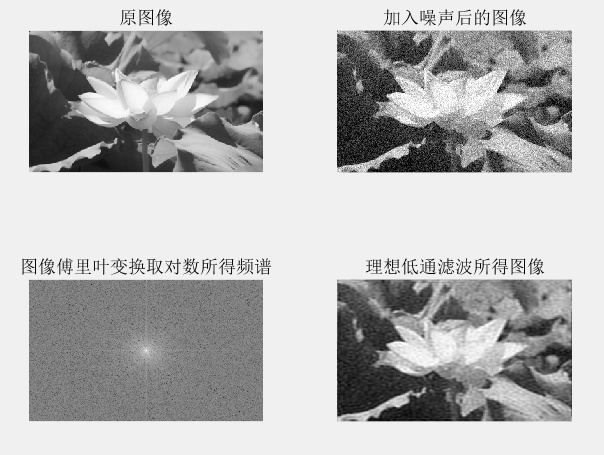#### 2 高斯低通滤波

%高斯低通
IA=imnoise(I,'gaussian');%%加入高斯白噪声
subplot(222),imshow(IA);
title('加入噪声后的图像');
[f1,f2]=freqspace(size(IA),'meshgrid');
D=100/size(IA,1);
r=f1.^2+f2.^2;
Hd=ones(size(IA));
for i=1:size(IA,1)
for j=1:size(IA,2)
t=r(i,j)/(D*D);
Hd(i,j)=exp(-t);
end
end
Y=fft2(double(IA));
Y=fftshift(Y);
Ya=Y.*Hd;
Ya=ifftshift(Ya);
Ia=real(ifft2(Ya));
subplot(221),imshow(uint8(I));
title('原图像');
subplot(223),imshow(uint8(Ia));
title('高斯低通滤波处理');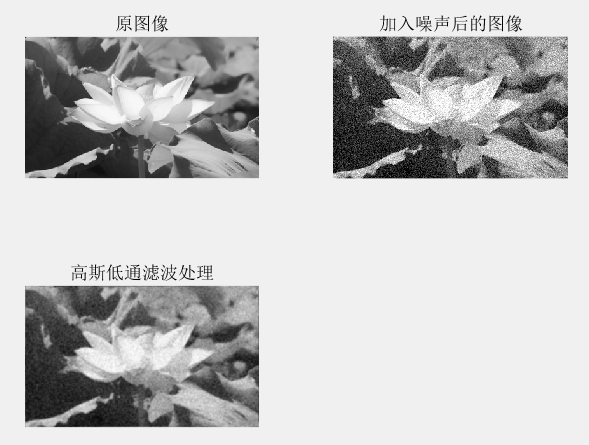#### 3 巴特沃斯低通滤波

clear all;
%巴特沃斯低通
figure(2);
subplot(221),imshow(I);
title('原始图像');
I1=imnoise(I,'gaussian');%%加入高斯白噪声
subplot(222),imshow(I1);
title('加入噪声后的图像');
f=double(I1);%数据类型转换
g=fft2(f);%图像傅里叶转换
g=fftshift(g);%傅里叶变换平移
F2=log(abs(g));%对傅里叶变换结果取绝对值，然后取对数
[N1,N2]=size(g);%傅里叶变换图像尺寸
n=2;%参数赋初始值
d0=30;
n1=fix(N1/2);%数据圆整
n2=fix(N2/2);%数据圆整
for i=1:N1%遍历图像像素
for j=1:N2
d=sqrt((i-n1)^2+(j-n2)^2);
if d==0
h=0;
else
h=1/(1+(d/d0)^(2*n));
end
result(i,j)=h*g(i,j);%?图像矩阵计算处理
end
end
F3=log(abs(result));%对傅里叶变换结果取绝对值，然后取对数
result=ifftshift(result);
X2=ifft2(result);
X3=uint8(real(X2));
subplot(223),imshow(uint8(X3))
title('Butterworth低通滤波图像');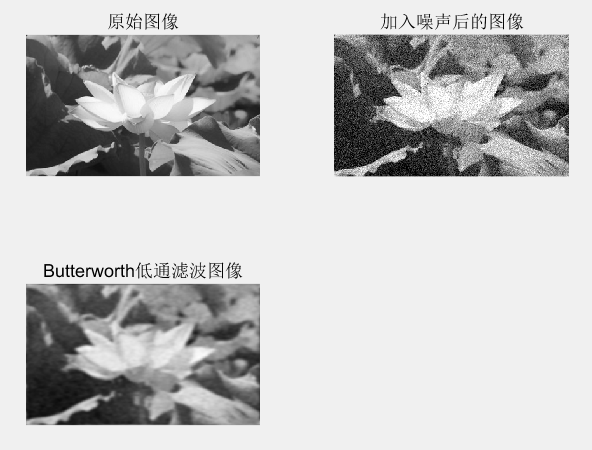展开全文• 巴特沃斯低通滤波matlab实现代码 Activity-Recognition-v2 Multi-classification problem, BP Neural Network, matlab 1.核心代码1：自行实现 预处理过程： Fs = 100; % 采样率 Wc=2*10/Fs; % 截止频率 [b,a]=butter...
• 我们已经介绍了频域中三种类型的低通滤波的代码； 1.理想低通滤波器（ILPF）（问题？） 2. 巴特沃斯低通滤波器 (BLPF) 3. 高斯低通滤波器（GLPF） 您可以清楚地观察到低通滤波器输出中的振铃效应问题。 振铃效应的...
• matlab低通滤波滤波，用于图像处理与分析，源代码，希望有帮助
• ## matlab巴特沃斯低通滤波器

万次阅读 多人点赞 2019-10-28 11:06:21
此处是MATLAB 的代码 wp=2*pi*5000;ws=2*pi*12000;Rp=2;Rs=30; [N,wc]=buttord(wp,ws,Rp,Rs,'s');% s is the anology filter [B,A]=butter(N,wc,'s'); sk=0:511; fk=0:14000/512:14000;wk=2*pi*fk; Hk=freqs(B,A,...

此处是MATLAB 的代码

wp=2*pi*5000;ws=2*pi*12000;Rp=2;Rs=30;
[N,wc]=buttord(wp,ws,Rp,Rs,'s');% s is the anology filter
[B,A]=butter(N,wc,'s');
sk=0:511; fk=0:14000/512:14000;wk=2*pi*fk;
Hk=freqs(B,A,wk);
plot(fk/1000,20*log10(abs(Hk)),'r') ;hold on
xlabel('频率(khz)');ylabel('幅度（db）');
axis([0,14,-40,5]);

[N,wc]=buttord(wp,ws,10,Rs,'s');% s is the anology filter
[B,A]=butter(N,wc,'s');
Hk=freqs(B,A,wk);
plot(fk/1000,20*log10(abs(Hk)),'g');

[N,wc]=buttord(wp,ws,20,Rs,'s');% s is the anology filter
[B,A]=butter(N,wc,'s');
Hk=freqs(B,A,wk);
plot(fk/1000,20*log10(abs(Hk)),'b');

legend('Rp=2','Rp=10','Rp=20','Location','northeast','Orientation','horizontal')


运行结果： 分析，Rp 越大，通达的振幅越大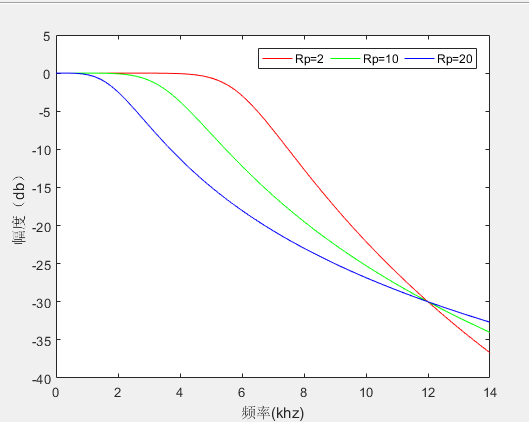参考“：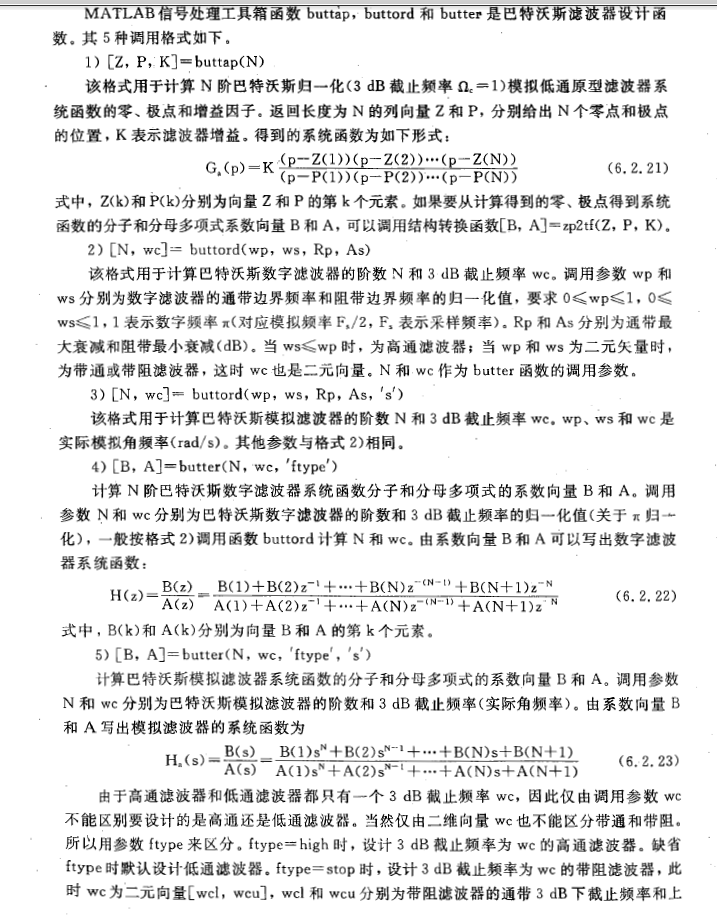展开全文• 这是matlab编写的butter worth低通滤波器，可以给学习这个方的朋友一个参考
• ## 用matlab做巴特沃斯低通滤波器

万次阅读 多人点赞 2020-07-11 23:00:39
matlab巴特沃斯低通滤波器的干货 基本数据 fs=100 N=1024 n=-N/2:N/2-1 f=n*fs/N也可以表示为f=linspace(-fs/2,fs/2,N); t=n/fs 第一步：做出原信号的频谱函数 注意：用fft函数作频谱分析，得到的是0~fs内的频谱...

# 用matlab做巴特沃斯低通滤波器

趁着暑假，做一个心电图的matlab实验，遇到了滤波器问题，网上代码比较杂乱，做了一个汇总整理。
主要做了一个简单的低通滤波器并以三角函数为例子进行低通滤波。

## 基本数据

fk=100  %采样频率
N=1024  %采样个数
n=-N/2:N/2-1
f=n*fk/N
t=n/fk
y=sin(2*pi*10*t)


当然 f也可以表示成 f=linspace(-fk/2,fk/2,N);

# 第一步：做出原信号的频谱函数

注意：用fft函数作频谱分析，得到的是0~fk内的频谱
而用fftshift函数得到-fk/2~fk/2内的频谱

fft_y=fft(y);
fftshift_y=fftshift(fft_y);
f=linspace(-50,50,1024);
plot(f,abs(fftshift_y));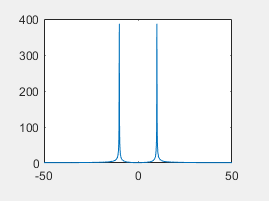## 第二步：做出巴特沃斯低通滤波器

### 用buttord函数求出阶数以及Wn

butter函数是求Butterworth数字滤波器的系数，在求出系数后对信号进行滤波时用filter函数
[g,Wn]=buttord(Wp,ws,Rp,Rs);
Wp=fp/(0.5fk)=20/50=0.4(通带截至频率)
Ws=fs/(0.5*fk)=30/50=0.6(阻带截止频率)
Rp=1; 通带最大衰减
Rs=30; 阻带最小衰减
代码如下：

[g,Wn]=buttord(0.4,0.6,1,30);


### 用butter 求出差分方程的系数b a

不懂差分方程及filter用法的朋友可以点以下链接，参考这位大佬的理解
差分方程介绍

[b,a]=butter(g,Wn);


至此 准备工作都做完了 ，来看看这滤波器的相频特性啥样子

### 滤波器的相频特性

 [q,w]=freqz(b,a,256);
plot(w*fs/(2*pi),abs(q))


所以说 咱写出来的滤波器长这个样子
20Hz为通带截至频率
30Hz为阻带截至频率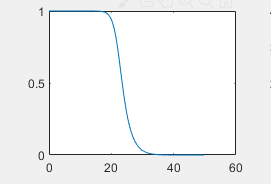## 第三步：用滤波器过滤信号 并得出频谱图

### 用filter过滤

用filter函数就能把原信号y过滤成k函数 ，需要注意的是，它们都是时域函数，需要进行fft变换才能看到频谱图

 k=filter(b,a,y);
fft_k=fft(k);
fftshift_k=fftshift(fft_k);
plot(f,abs(fftshift_k));


过滤后的结果如下：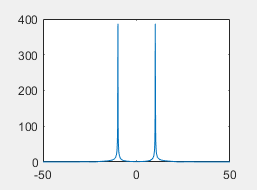没错，没变！我们低通滤波器允许20Hz以下的信号不变，而我们原信号的频率就是10Hz！

### 对高频的信号的低通滤波

这里就不赘述了，对y=sin(2pi40*t)的信号，依然用上面的低通过滤的结果如下图：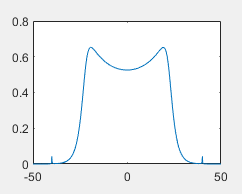不要看它长的怪，注意一下幅值，约等于0，即把40Hz的信号过滤掉了！
行文至此，点个赞呗亲！

展开全文filter 过滤器
• 基于Matlab巴特沃斯低通滤波器的设计谢继杨(成都理工大学工程技术学院，四川乐山，614000)摘要：现如今已经有相当成熟的技术去模拟滤波器，人们为了更加深入的理解巴特沃斯滤波器，于是巴特沃斯模拟滤波器便基于...
• 基于matlab虹膜图像高斯滤波、低通滤波巴特沃斯滤波 二、源代码 function varargout = frequencydem(varargin) % Begin initialization code - DO NOT EDIT gui_Singleton = 1; gui_State = struct('gui_Name', ...
• MATLAB：去除信号噪音 代码： %读音频test，添加噪声后滤除噪声 clear;clc; [audio,fs]=audioread('test.wav');%声音读取audio是读出数据 fs为音频文件的采样率 audio = audio(:,1); %双通道变单通道 n=length(audio...
• title('巴特沃斯高通滤波图像'); function im5 = freqfilter_btw_Hp(im,D0,n) % 巴特沃斯高通滤波器 % input: % M,N:频域滤波器的尺寸 % D0：带阻滤波器的截止频率 % n ：阶次 % output: % H：M x N的矩阵，表示...
• 求教用c语言实现IIR巴特沃斯低通滤波器效果实验数据：x=[-0.0289, 0.3943, 0.9965, 1.1266, 0.9995, 1.0891, 1.2262, 1.0699,...0.8990, 0.7685, 0.7844, 0.9471, 1.4317, 1.6765, 1.7629,-3.2903,...3.4122, 4.5403...
• 巴特沃斯滤波器滤波器例程，可通过修改程序实现巴特沃斯高通滤波器和低通滤波器、调节阶数，内含实验图像。典型的巴特沃斯滤波器例程。
• 利用巴特沃斯滤波器对加噪图像进行滤波处理。 处理的一些思路： 实在是不得不感慨MATLAB的强大。实验很简单。基本就是依靠的仿真软件。 对图像进行加噪处理直接调用了现成的函数。 对图像的滤波处理。将图片的数据...
• function filter_img = Lowpass_filter...%--巴特沃斯低通滤波器 I = input_img; I = im2double(I); N = 2*size(I,1); M = 2*size(I,2); u = -M/2:(M/2-1); v = -N/2:(N/2-1); [U,V] = meshgrid(u,v); D = sqrt...
• 基于Matlab巴特沃斯低通滤波器的设计谢继杨(成都理工大学工程技术学院，四川乐山，614000)摘要：现如今已经有相当成熟的技术去模拟滤波器，人们为了更加深入的理解巴特沃斯滤波器，于是巴特沃斯模拟滤波器便基于...
• 基于MATLAB巴特沃斯低通滤波器.基于MATLAB设计巴特沃斯低通滤波器课程设计专 业： XXXXXX姓 名： XXX学 号： XX指导老师： XXX2011年 11 月 26日通信系统仿真课程设计任务书院(系)：电气信息工程学院姓名XXX学号XX...
• 数字图像处理，评语滤波，理想低通滤波器，高斯滤波器，巴特沃斯滤波器数字图像处理
• 建议参考书籍：数字图像处理 高斯高/低通滤波 低通滤波： 在这里插入代码片 高通滤波器： 在这里插入代码片
• ## matlab低通滤波

万次阅读 多人点赞 2018-04-16 21:02:49
所以低通滤波的效果是图像去噪声平滑增强，但同时也抑制了图像的边界，造成图像不同程度上的模糊。大于大小为M×N的图像，频率点（u，v）与频率中心的距离为D（u，v），其表达式为：1. 理想低通滤波器产生公式为：...
• 利用matlab设计巴特沃斯低通滤波器 三峡大学 课 程 设 计 报 告 专业班级 20091421 课 程 数字信号处理课程设计 学 号 2009142116 学生姓名 姜祥奔 指导教师 王露 2012年 5 月 平时成绩(20%) 报告成绩(40%) 答辩成绩......

# matlab巴特沃斯低通滤波matlab 订阅